AVL 树

一：什么是 AVL 树？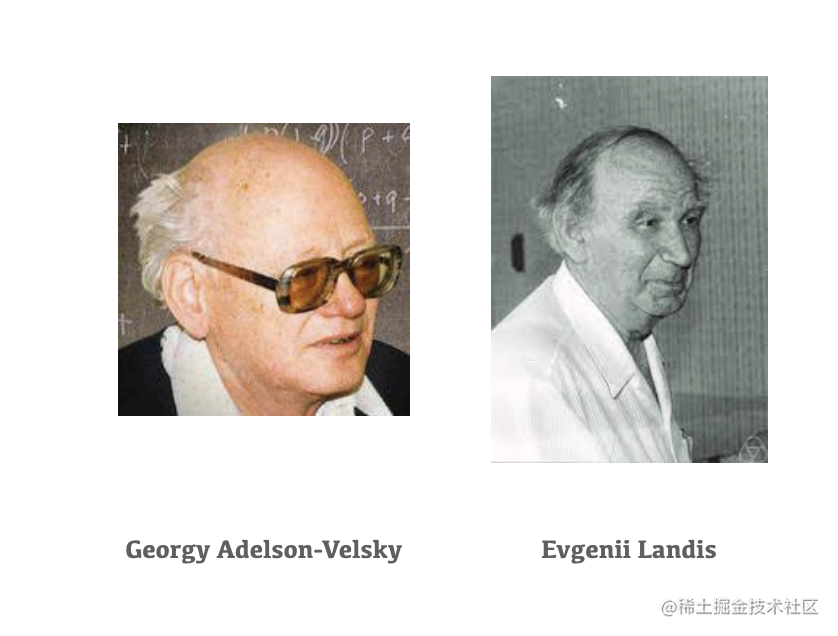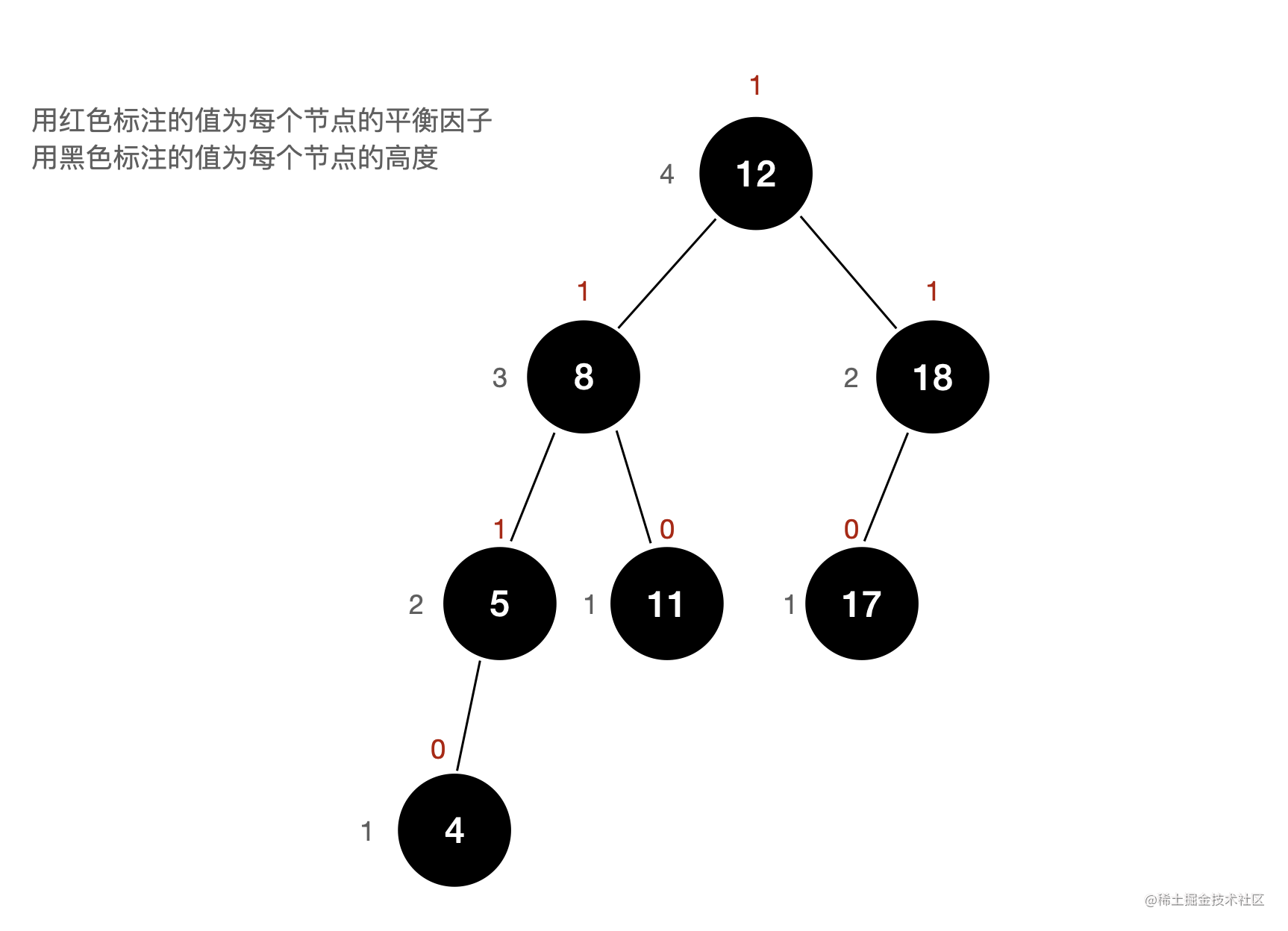二：AVL 树的实现

1. 节点高度与平衡因子

AVL 树是如何实现自平衡的？

AVL 想要满足平衡二叉树的这个特性，就要保证所有节点的平衡因子的绝对值不超过 1。所以，AVL 树能够做到维持自平衡，实际上就是在树的结构发生变化时（向 AVL 中添加节点或删除节点），通过检测节点的平衡因子来调整树的结构。

AVL 树的节点除了存储左孩子与右孩子的引用以及自身的 value 值以外，还需要能够表示出当前节点的高度。

public class Node<E extends Comparable<E>> {
public E e;
public Node left;
public Node right;
public int height;

public Node(E e) {
this.e = e;
left = null;
right = null;
height = 1;
}
}

/**
* 返回 node 节点的高度
*
* @param node
* @return
*/
private int getHeight(Node node) {
if (node == null)
return 0;
return node.height;
}

/**
* 获得节点 node 的平衡因子
*
* @param node
* @return
*/
private int getBalanceFactor(Node node) {
if (node == null)
return 0;
return getHeight(node.left) - getHeight(node.right);
}

2. 向 AVL 树中添加元素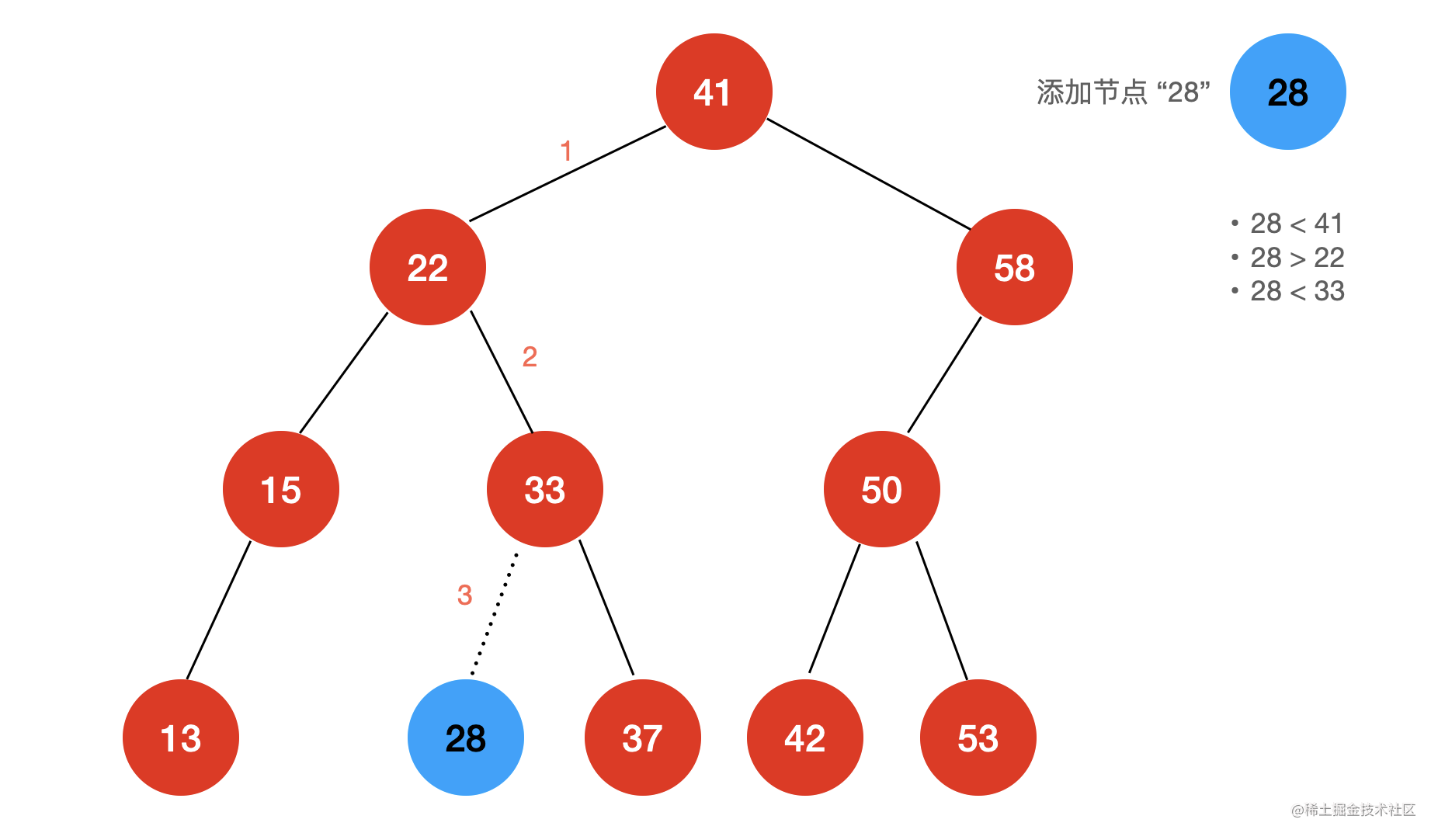Java 代码如下：

/**
* @param e 向二分搜索树中添加新的元素
*/
}

/**
* @param e    向二分搜索树中新插入的节点
* @param node 当前比较的节点
* @return 返回二分搜索树的根节点
*/
private Node add(E e, Node node) {
if (node == null) {
size++;
return new Node(e);
}
if (e.compareTo((E) node.e) < 0) {
} else if (e.compareTo((E) node.e) > 0) {
}
return node;
}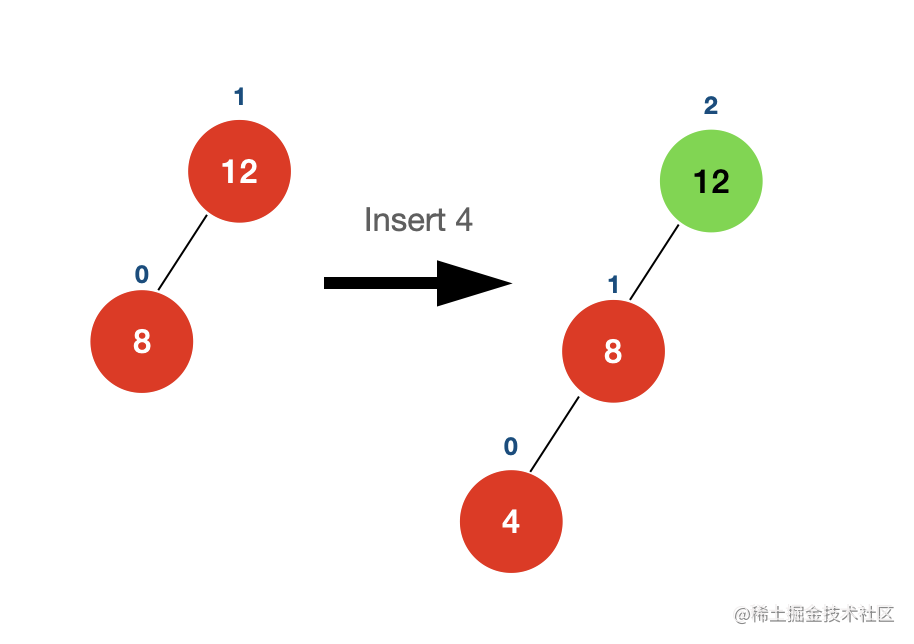getBalanceFactor(node) > 1 && getBalanceFactor(node.left) >= 0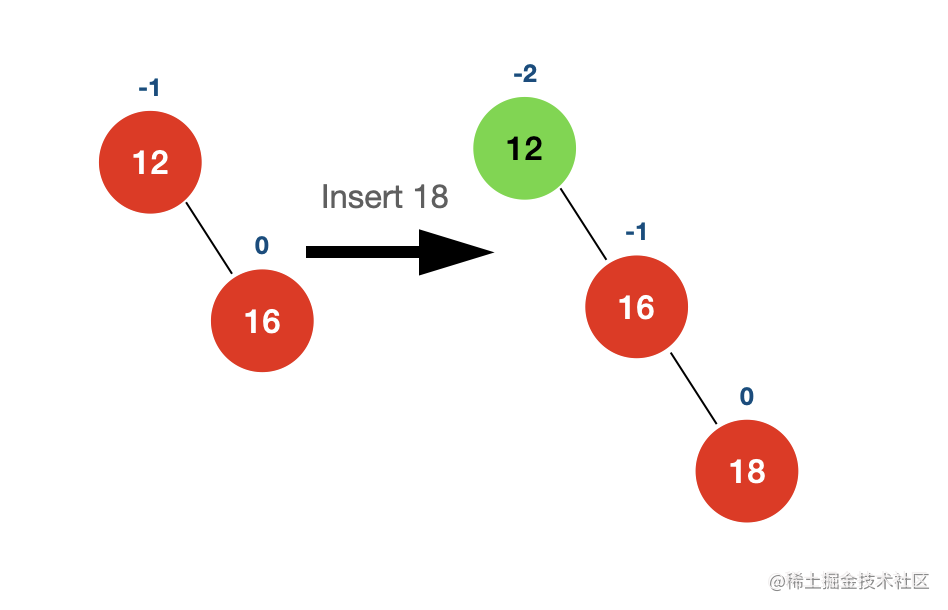getBalanceFactor(node) < -1 && getBalanceFactor(node.right) <= 0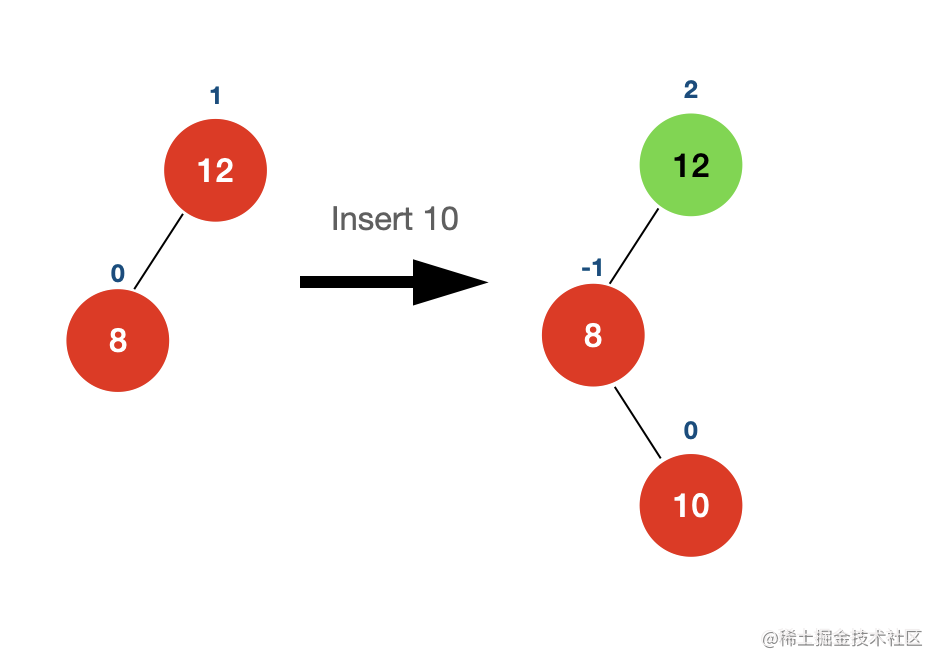getBalanceFactor(node) > 1 && getBalanceFactor(node.left) < 0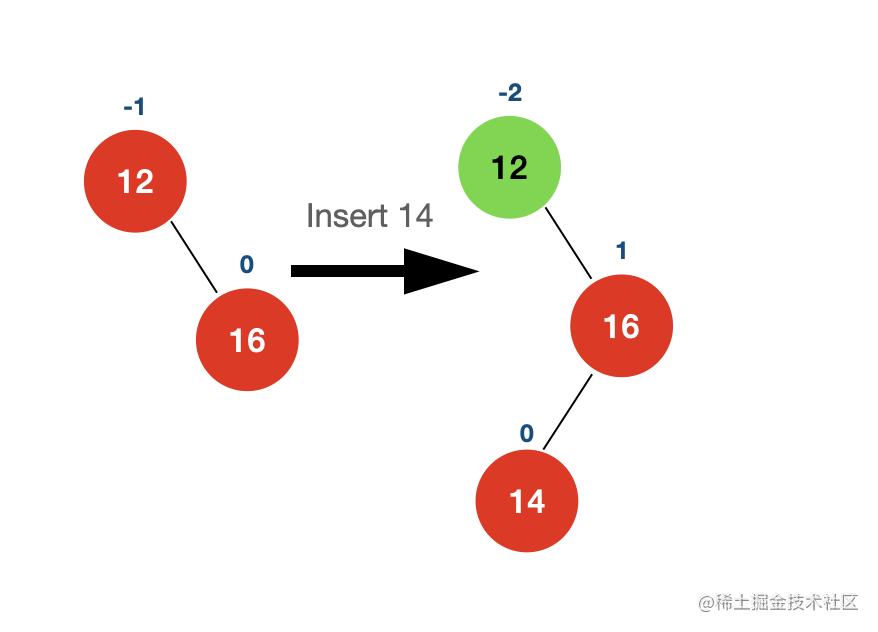getBalanceFactor(node) < -1 && getBalanceFactor(node.right) > 0

左旋与右旋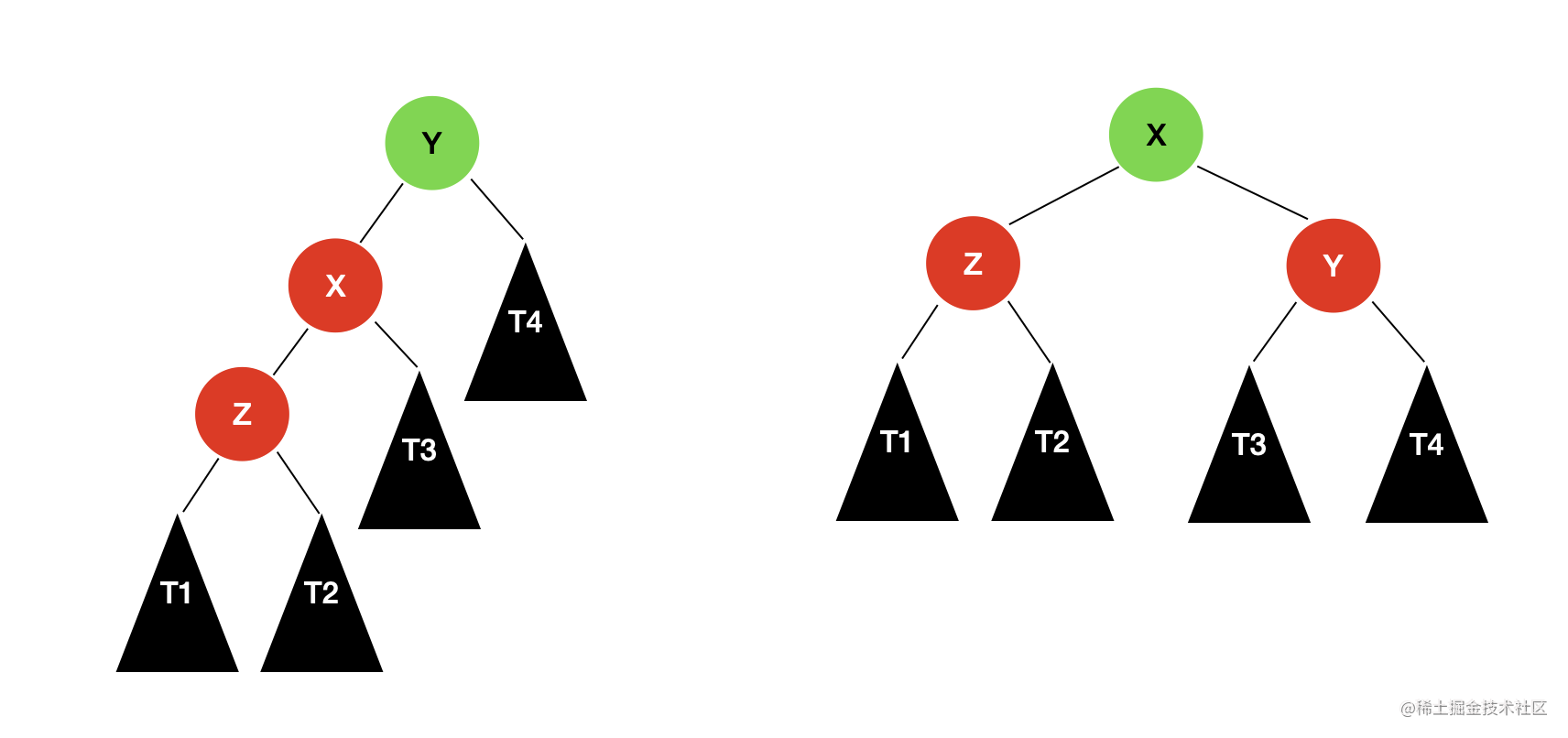$T1$$T2$ 中，最大高度为 $h$，所以节点 $z$ 的高度为 $h+1$；节点 $x$ 也是平衡的，其平衡因子大于等于 0 且小于等于 1；所以，$T3$ 的高度不是 $h+1$ 就是 $h$$x$ 的高度表示为 $h+2$$y$ 节点是不平衡的，它的平衡因子一定是 2，所以，$T4$ 的高度为 $h$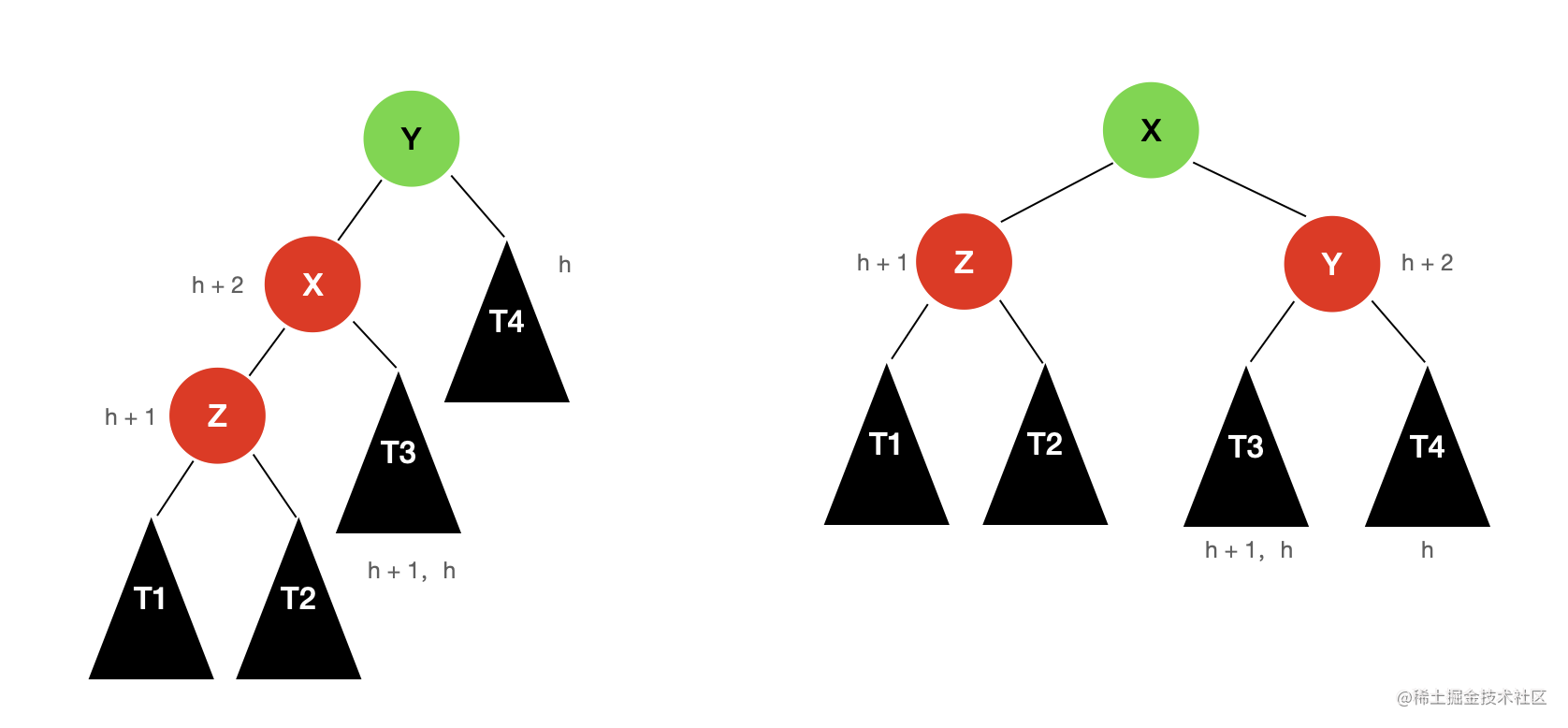/**
* 右旋:
*
*            y                           x
*          /  \                       /     \
*        x     T4                    z        y
*      /  \         ========>     /  \      /  \
*    z     T3                   T1   T2   T3   T4
*  /  \
* T1  T2
*/
private Node rightRotate(Node y){
Node x = y.left;
Node T3 = x.right;
x.right = y;
y.left = T3;

// 更新 height
y.height = Math.max(getHeight(y.left),getHeight(y.right)) + 1;
x.height = Math.max(getHeight(x.left),getHeight(x.right)) + 1;
return x;
}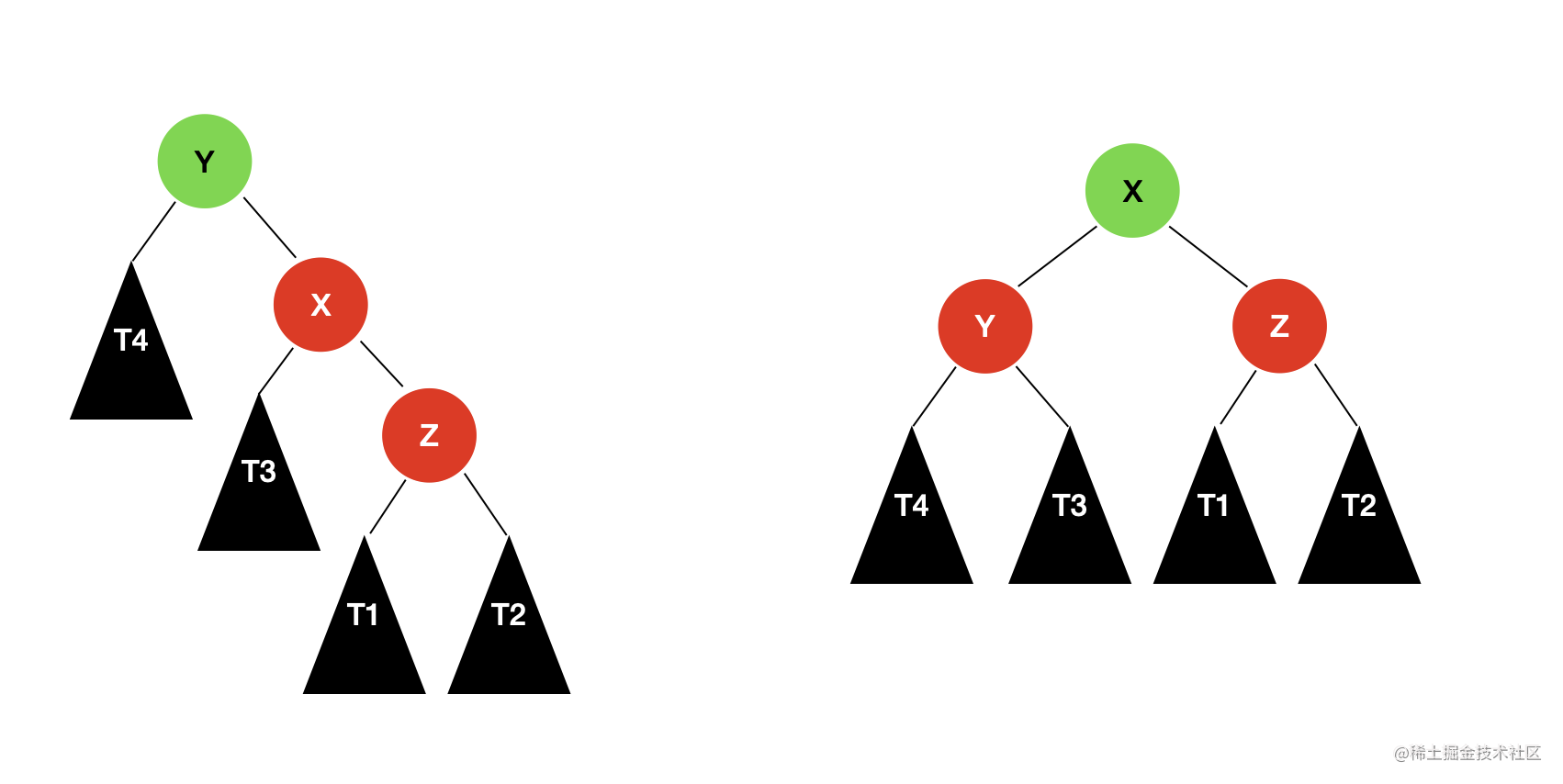/**
* 左旋:
*
*            y                           x
*          /  \                       /     \
*        T4    x                    y         z
*             /  \    ========>   /  \      /  \
*            T3   z             T4   T3   T1   T2
*               /  \
*              T1  T2
*/
private Node leftRotate(Node y){
Node x = y.right;
Node T3 = x.left;
x.left = y;
y.right = T3;

// 更新 height
y.height = Math.max(getHeight(y.left),getHeight(y.right)) + 1;
x.height = Math.max(getHeight(x.left),getHeight(x.right)) + 1;

return x;
}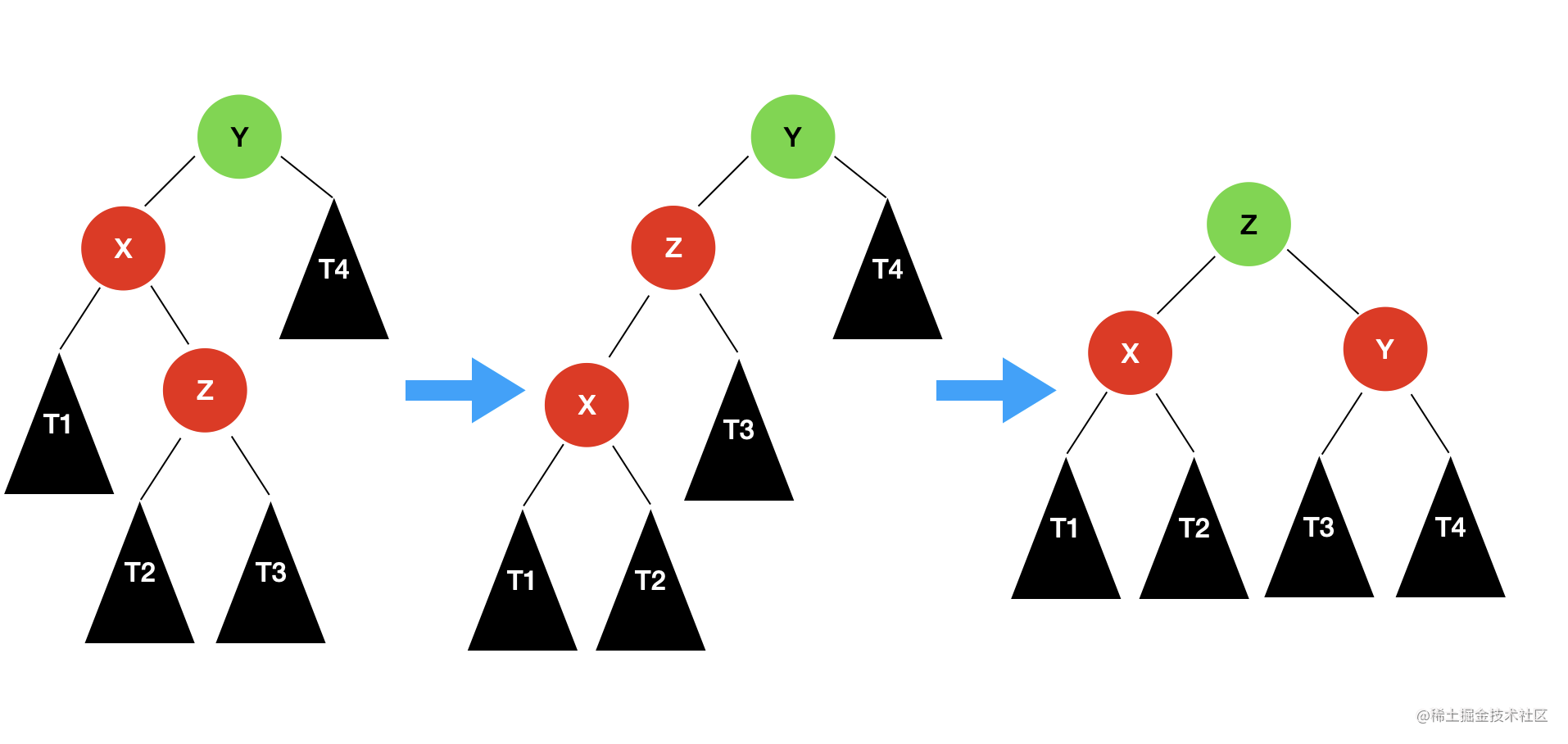向 AVL 树中添加元素的完整代码

/**
* @param e 向 AVL 中添加新的元素
*/
}

/**
* @param e    向 AVL 中新插入的节点
* @param node 当前比较的节点
* @return 返回 AVL 的根节点
*/
private Node add(E e, Node node) {
if (node == null) {
size++;
return new Node(e);
}
if (e.compareTo((E) node.e) < 0) {
} else if (e.compareTo((E) node.e) > 0) {
}

// 更新 height
node.height = 1 + Math.max(getHeight(node.left), getHeight(node.right));

// 计算平衡因子
int balanceFactor = getBalanceFactor(node);

// 平衡的维护
// LL
if (balanceFactor > 1 && getBalanceFactor(node.left) >= 0)
return rightRotate(node);

// RR
if(balanceFactor < -1 && getBalanceFactor(node.right) <= 0)
return leftRotate(node);

// LR
if(balanceFactor > 1 && getBalanceFactor(node.left) < 0) {
node.left = leftRotate(node.left);
return rightRotate(node);
}

// RL
if(balanceFactor < -1 && getBalanceFactor(node.right) > 0) {
node.right = rightRotate(node.right);
return leftRotate(node);
}
return node;
}

3. 向 AVL 树中删除元素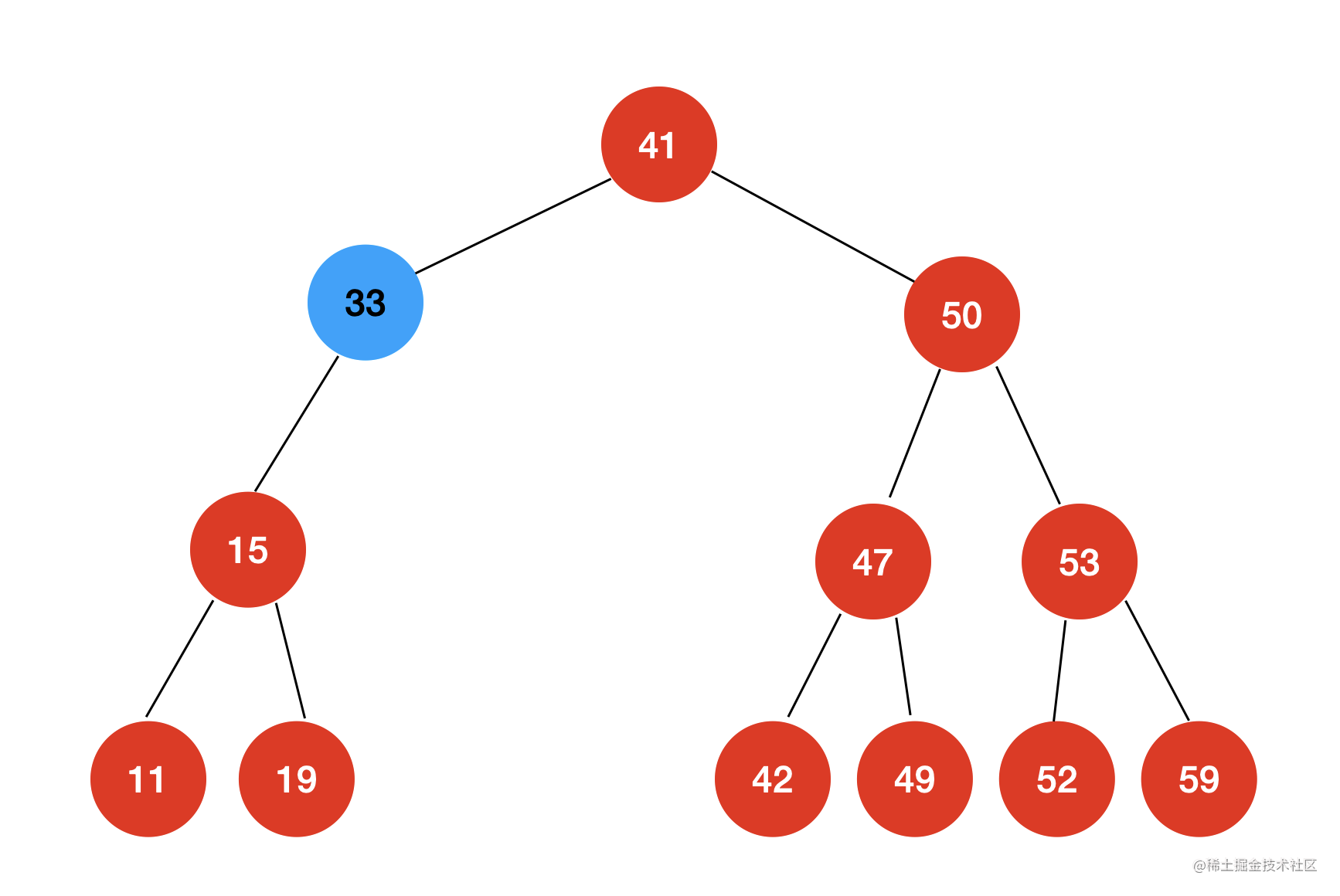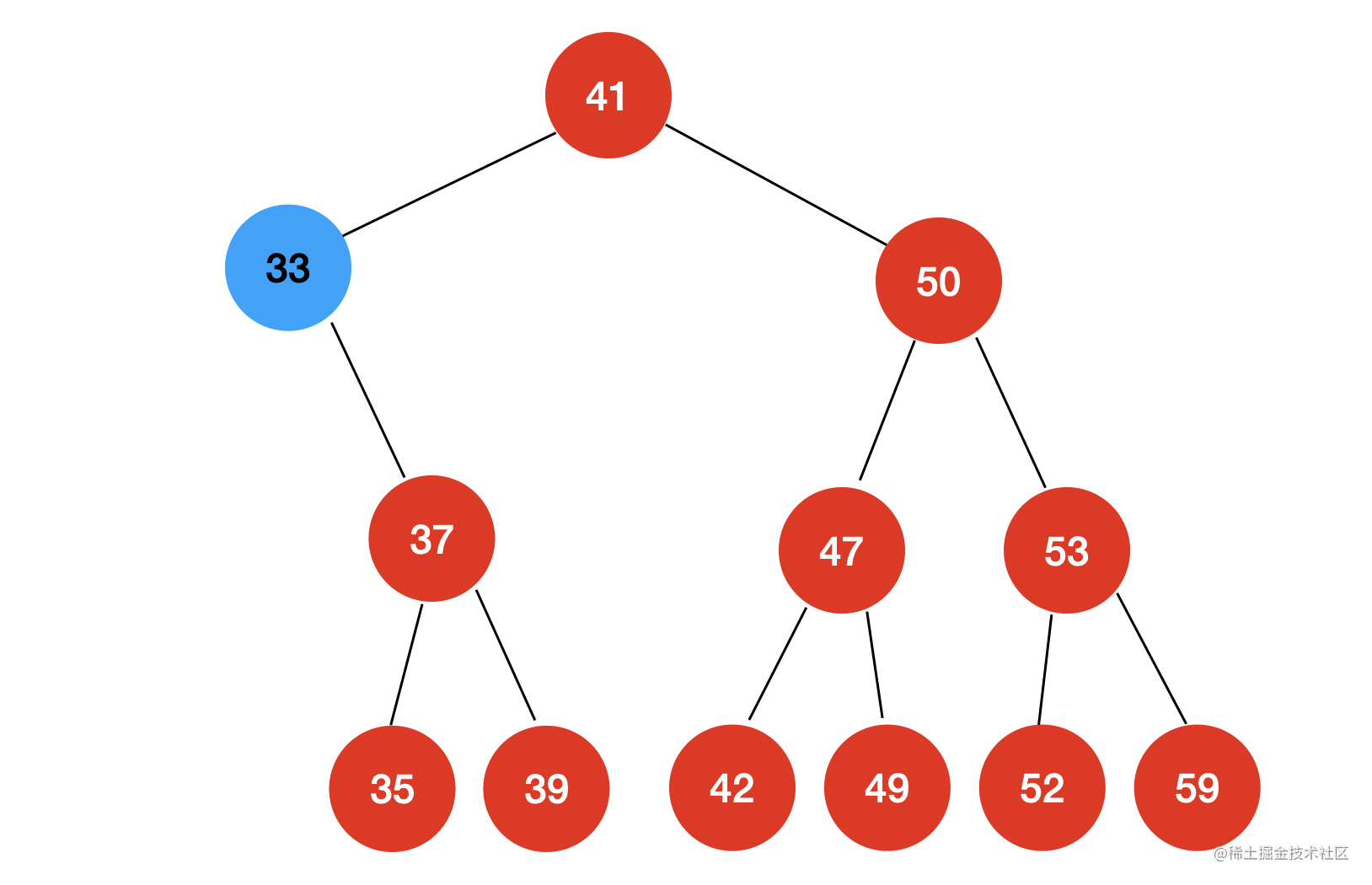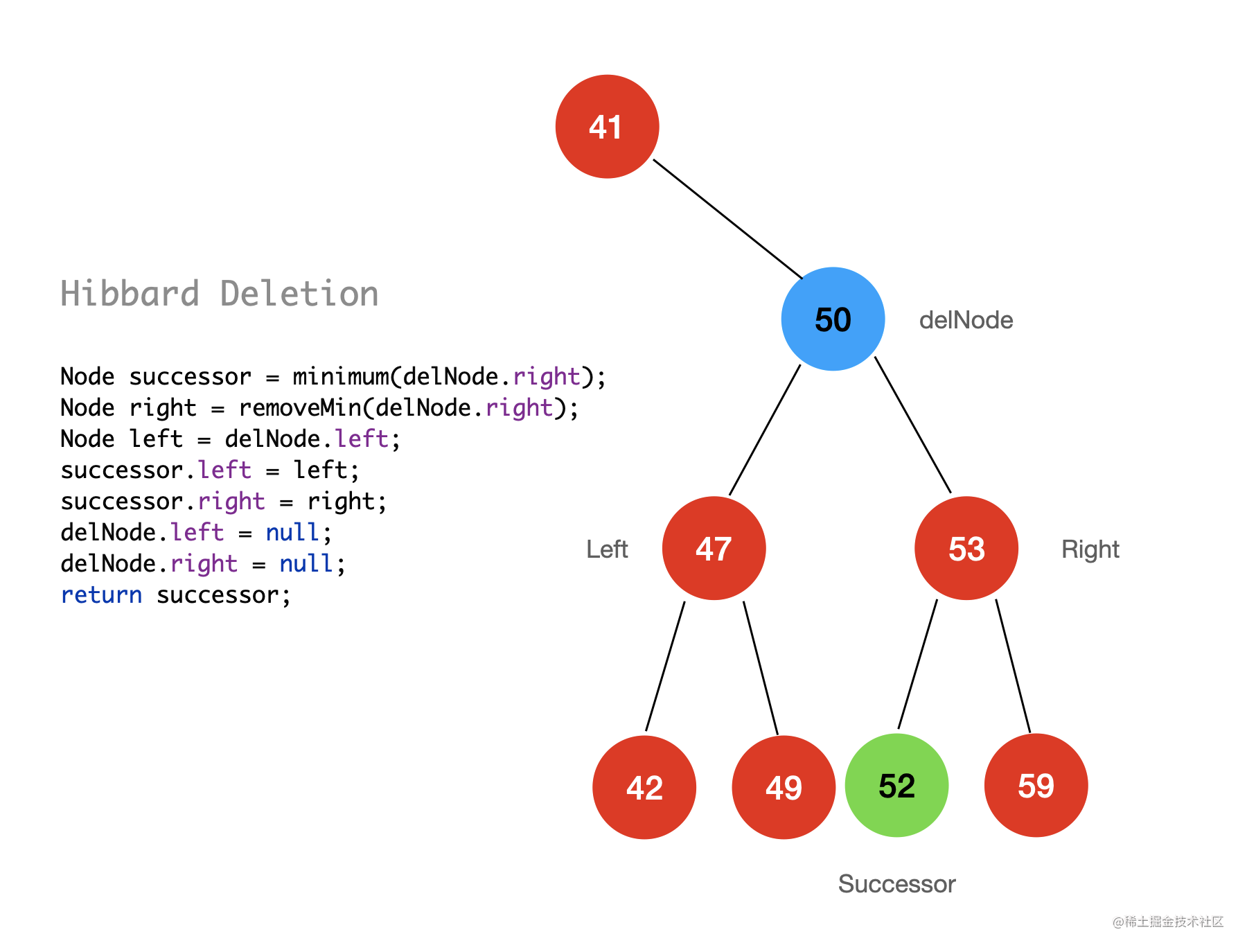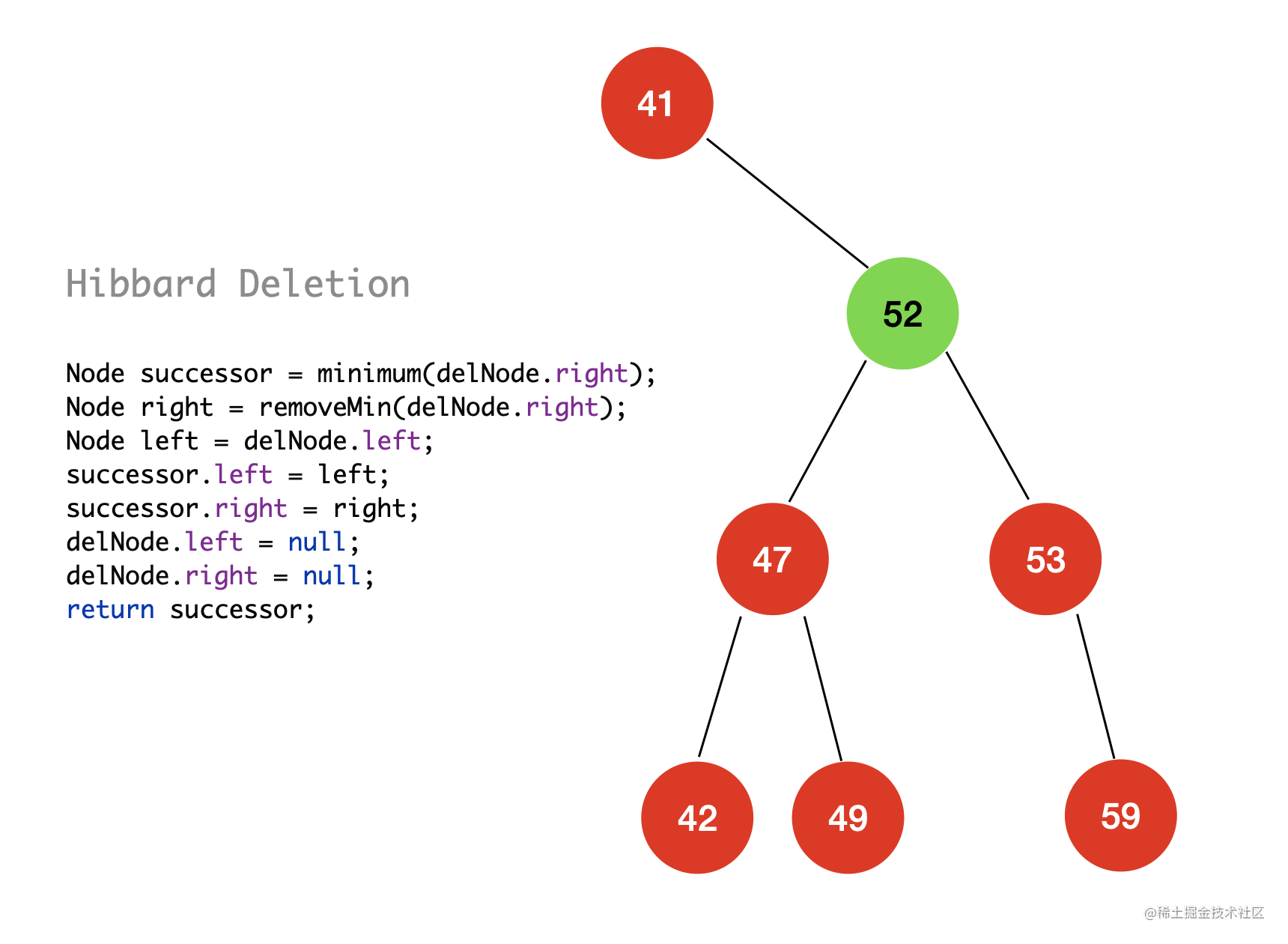public void remove(E e) {
root = remove(e, root);
}

private Node remove(E e, Node node) {
if (node == null) {
return null;
}
if (e.compareTo((E) node.e) < 0) {
node.left = remove(e, node.left);
return node;
} else if (e.compareTo((E) node.e) > 0) {
node.right = remove(e, node.right);
return node;
} else {
if (node.left == null) {
Node rightNode = node.right;
node.right = null;
size--;
return rightNode;
}
if (node.right == null) {
Node leftNode = node.left;
node.left = null;
size--;
return leftNode;
}

// Hibbard Deletion
Node successor = minimum(node.right);
Node right = removeMin(node.right);
Node left = node.left;
successor.left = left;
successor.right = right;
node.left = null;
node.right = null;
return successor;
}
}

public void remove(E e) {
root = remove(e, root);
}

private Node remove(E e, Node node) {
if (node == null)
return null;

Node retNode;
if (e.compareTo((E) node.e) < 0) {
node.left = remove(e, node.left);
retNode = node;
} else if (e.compareTo((E) node.e) > 0) {
node.right = remove(e, node.right);
retNode = node;
} else {
// 待删除节点的左子树为空
if (node.left == null) {
Node rightNode = node.right;
node.right = null;
size--;
retNode = rightNode;
}
// 待删除的节点右子树为空时
else if (node.right == null) {
Node leftNode = node.left;
node.left = null;
size--;
retNode = leftNode;
}
// 待删除的节点左右子树均不为空
else {// Hibbard Deletion
Node successor = minimum(node.right);
Node right = remove((E) node.right, successor);
Node left = node.left;
successor.left = left;
successor.right = right;
node.left = null;
node.right = null;
retNode = successor;
}
}

if(retNode == null)
return null;

// 更新 height
retNode.height = 1 + Math.max(getHeight(retNode.left), getHeight(retNode.right));

// 计算平衡因子
int balanceFactor = getBalanceFactor(retNode);

// 平衡的维护

// LL
if (balanceFactor > 1 && getBalanceFactor(retNode.left) >= 0)
return rightRotate(retNode);

// RR
if(balanceFactor < -1 && getBalanceFactor(retNode.right) <= 0)
return leftRotate(retNode);

// LR
if(balanceFactor > 1 && getBalanceFactor(retNode.left) < 0) {
retNode.left = leftRotate(retNode.left);
return rightRotate(retNode);
}

// RL
if(balanceFactor < -1 && getBalanceFactor(retNode.right) > 0) {
retNode.right = rightRotate(retNode.right);
return leftRotate(retNode);
}
return retNode;
}

三：AVL 树与二分搜索树的性能测试

/**
* 验证当前的 AVL 树是否满足二分搜索树的特性
*
* @return
*/
public boolean isBST() {
ArrayList<E> list = new ArrayList<>();
inOrder(root, list);
for (int i = 1; i < list.size(); i++)
if (list.get(i - 1).compareTo(list.get(i)) > 0)
return false;
return true;
}

/**
* @param node 中序遍历以 node 为 根的 AVL
*/
private void inOrder(Node node, List<E> list) {
if (node == null) {
return;
}
inOrder(node.left, list);
inOrder(node.right, list);
}

/**
* 验证当前的二叉树是否是一棵平衡的二叉树
*
* @return
*/
public boolean isBalanced() {
return isBalanced(root);
}

/**
* 验证当前的二叉树是否是一棵平衡的二叉树
*
* @param node
* @return
*/
private boolean isBalanced(Node node) {
if (node == null)
return true;
int balanceFactor = getBalanceFactor(node);
if (Math.abs(balanceFactor) > 1)
return false;
return isBalanced(node.left) && isBalanced(node.right);
}

class AVLTreeTest {

@Test
void AVLAndBSTPerformanceTest() {
int[] testArr = new int;
for (int i = 0; i < testArr.length; i++)
testArr[i] = i;
testBst(testArr);
testAvl(testArr);
}

@Test
void AVLTest(){
AVLTree<Integer> avl = new AVLTree<>();
for (int i = 0; i < 1000; i++){
if(!avl.isBalanced() || !avl.isBST())
throw new RuntimeException("error!");
}
for(int i = 0; i < 1000; i++){
avl.remove(i);
if(!avl.isBalanced() || !avl.isBST())
throw new RuntimeException("error!");
}
System.out.println("correct!");

}

private void testBst(int[] testArr) {
BST<Integer> bst = new BST<>();
long start = System.nanoTime();
for (int i = 0; i < testArr.length; i++)
for (int i = 0; i < testArr.length; i++)
if (!bst.contains(i)) throw new RuntimeException("error");
long end = System.nanoTime();
System.out.println("BST : " + (end - start) / 1000000000.0 + " s");
}

private void testAvl(int[] testArr) {
AVLTree<Integer> avl = new AVLTree<>();
long start = System.nanoTime();
for (int i = 0; i < testArr.length; i++)
for (int i = 0; i < testArr.length; i++)
if (!avl.contains(i)) throw new RuntimeException("error");
long end = System.nanoTime();
System.out.println("AVL : " + (end - start) / 1000000000.0 + " s");
}
}

BST : 1.935773389 s
AVL : 0.018965793 s

四：总结

AVL 树是计算机科学中，最早被发明的自平衡二叉查找树，除了 AVL 外，还有很多优秀的平衡二叉查找树这种数据结构，期待在后面的文章中，我可以与你一同研究与学习。

github.com/jinrunheng/…